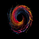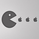7783 views
Wave Channel 3D
Built by Ricardo idea from JR & Aloakdutt from indieTrades Jan. 2010

This indicator is very easy to build. We utilize Moving Averages with a set multiplier and an offset. Specially we try to use Fibonacci sequence series numbers (1, 2, 3, 5, 8, 13, 21, 34, 55, 89, 144...) as time space and multiplier (default 89, 8). Also included is Donchian Channel to locate strong trends and possible future support - resistance.

Examples of support/resistance on chart.

Dominant Price Trends

Future Support Resistance

Comparing Fibonacci Series Time Space - Multiplier

When Comparing make note of confluence support/resistance showing up with Fibonacci Series
Example uses DC

When Comparing make note of confluence support/resistance showing up with Fibonacci Series
Example without DC / Smooth MA
```study(title="3D-Wave Channel", shorttitle="3D-WC", overlay=true)
timespace = input(1)
smooth = input(89)
offsetMultiplier=input(8)
useDonchianAverage = input(false)
src = input(ohlc4)
ma = useDonchianAverage ? avg(highest(smooth),lowest(smooth)) : ema(src, smooth)
plot(ma, color=black, offset=offsetMultiplier*01)
plot(ma[timespace*01], color=silver, offset=offsetMultiplier*02)
plot(ma[timespace*02], color=silver, offset=offsetMultiplier*03)
plot(ma[timespace*03], color=gray, offset=offsetMultiplier*04)
plot(ma[timespace*04], color=gray, offset=offsetMultiplier*05)
plot(ma[timespace*05], color=gray, offset=offsetMultiplier*06)
plot(ma[timespace*06], color=silver, offset=offsetMultiplier*07)
plot(ma[timespace*07], color=silver, offset=offsetMultiplier*08)
plot(ma[timespace*08], color=gray, offset=offsetMultiplier*09)
plot(ma[timespace*09], color=gray, offset=offsetMultiplier*10)
plot(ma[timespace*10], color=black, offset=offsetMultiplier*11)

max_ma()=>max(ma[timespace*10],max(ma[timespace*9],max(ma[timespace*8],max(ma[timespace*7],max(ma[timespace*6],max(ma[timespace*5],max(ma[timespace*4],max(ma[timespace*3],max(ma[timespace*2],max(ma[timespace*1], ma))))))))))
min_ma()=>min(ma[timespace*10],min(ma[timespace*9],min(ma[timespace*8],min(ma[timespace*7],min(ma[timespace*6],min(ma[timespace*5],min(ma[timespace*4],min(ma[timespace*3],min(ma[timespace*2],min(ma[timespace*1], ma))))))))))

top = highest(max_ma(), smooth)
bot = lowest(min_ma(), smooth)

plot(top, color=black, offset=offsetMultiplier)
plot(bot, color=black, offset=offsetMultiplier)```Thank you for sharing this interesting idea. The indicator initially seems to be really great based from the screenshot, but when I tested the signals they are lagging extremely behind the price movement. Even with very fast settings like smooth input(5), offsetMultiplier input(1) I could not find anything I would find useful in actual day-trading.

Don't get me wrong. I think this indicator is great when you analyze a new symbol for the very first time. It is very good to see the overall trend direction of recent weeks; to get an overall feeling where the market was headed recently.ChartArt
Thanks for that feedback ChartArt. Have explored all the features this indicator might provide. Current and future Support/Resistance , Trend Breakouts and more?QuantitativeExhaustion
My setting in action. Useful to see the overall strongest recent trend direction (and yes, also the major past support/resistance zones).ChartArt
Period 4 smoothed on weekly SPY chart. If only trading would be always that easy :DChartArt
You should also compare longer time series and multipliers. I picked Fibonacci Series, showing how in the example above. More support/resistance folds, higher probability of seeing those areas again displaying support/resistance.